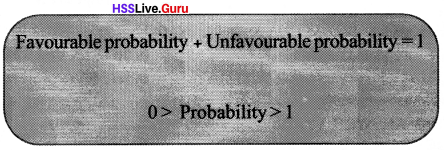# Kerala Syllabus 10th Standard Maths Solutions Chapter 3 Mathematics of Chance

## Kerala State Syllabus 10th Standard Maths Solutions Chapter 3 Mathematics of Chance

### Mathematics of Chance Text Book Questions and Answers

Textbook Page No. 71

Mathematics Of Chance Questions And Answers Kerala Syllabus 10th Standard Question 1.
A box contains 6 black and 4 white balls. If a ball is taken from it. What is the probability of it being black? And the probability of it being white?
Total no. of balls = 10
No. of black balls = 6
No. of white balls = 4
Probability of it to be black = $$\frac { 6 }{ 10 }$$ = $$\frac { 3 }{ 5 }$$
Probablitiy of to be white = $$\frac { 4 }{ 10 }$$ = $$\frac { 2 }{ 5 }$$

Mathematics Of Chance Class 10 Kerala Syllabus Kerala Syllabus Question 2.
There are 3 red balls and 7 green balls in a bag, 8 red and 7 green balls in another.
i. What is the probability of getting a red ball from the first bag?
ii. From the second bag?
iii. If all the balls are put in a single bag, What is the probability of getting a red ball from it?
i. Total no. of balls in the first bag = 10
No. of red balls = 3
P(red ball) = 3/10

ii. Total no. of balls in the second bag= 15 No. of red balls = 8
P(red ball) = 8/15

iii. Total no. of balls in both bags = 25
Total no. of red balls = 11
P(red ball) = 11/25

Mathematics Of Chance Questions And Answers Pdf Kerala Syllabus 10th Standard Question 3.
One is asked to say a two-digit number. What is the probability of it being a perfect square?
Total no. of two-digit numbers = 90
Perfect squares from 10 to 99 are 16, 25, 36, 49, 64, 81.
There are 6 favourable numbers Probability = $$\frac { 6 }{ 90 }$$ = $$\frac { 1 }{ 15 }$$

Mathematics Of Chance Extra Questions And Answers Kerala Syllabus 10th Standard Question 4.
Numbers from 1 to 50 are written on slips of paper and put in a box. A slip is to be drawn from it; but before doing so, one must make a guess about the number, either prime number or a multiple of five. Which is the better guess? Why?
Probablity of getting prime numbers from 1 to 50 = $$\frac { 15 }{ 50 }$$ = $$\frac { 3 }{ 10 }$$
Probability of getting a multiple of 5 from 1 to 50 = $$\frac { 10 }{ 50 }$$ = $$\frac { 1 }{ 5 }$$
∴ Better guess would be prime numbers as probability of that is more.

Sslc Maths Mathematics Of Chance Kerala Syllabus 10th Standard Question 5.
A bag contains 3 red beads and 7 green beads. Another contains one red and one green more. The probability of getting a red from which bag is more?
Total no. of beads in first bag = 10
No. of red beads in first bag = 3
P(red ball) in first bag = $$\frac { 3 }{ 10 }$$
Total no. of beads in second bag = 12
No. of red beads in second bag = 4
P(red ball) in second bag = $$\frac { 4 }{ 12 }$$ = $$\frac { 1 }{ 3 }$$
The probability of getting a red bead is more from the second bag since, $$\frac{1}{3}>\frac{3}{10}\left(\frac{10}{30}>\frac{9}{30}\right)$$

Textbook Page No. 72

In each picture below, the explanation of the green part is given. Calculate in each, the probability of a dot put without looking to be within the green part.

Mathematics Of Chance Pdf Kerala Syllabus 10th Standard Question 1.
A square got by joining the mid points of a bigger square.∴ Probability = $$\frac { 4 }{ 8 }$$ = $$\frac { 1 }{ 2 }$$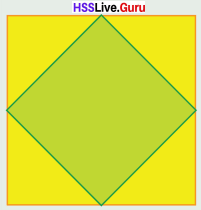Class 10 Maths Chapter 3 Mathematics Of Chance Kerala Syllabus Question 2.
A square with all vertices on a circleSide of square = a = 2cm Radiusie., The area of the square is 2/π the area of the circle.Probability = $$\frac{a^{2}}{\frac{\pi}{2} a^{2}}=\frac{4}{2 \pi}=\frac{2}{\pi}$$
Probablity ofthe dot falling on the square is $$\frac { 2 }{ π }$$ = 0.64

Sslc Maths Chapter 3 Questions And Answers Kerala Syllabus Question 3.
Circle exactly fitting inside a square.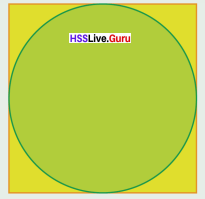If radius of circle is r
Side of the square = 2r
Area of the square = 4r2
Area of the circle= πr2Mathematics Of Chance Extra Questions Kerala Syllabus 10th Standard Question 4.
A triangle got by joining alternate vertices of a regular hexagon.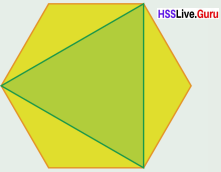The area of regular hexagon having side a = $$\frac{3 \sqrt{3}}{2} a^{2}$$
Side of the triangle got by joining alternate vertices = √3a
Area of triangle = $$\frac{\sqrt{3}}{4}(\sqrt{3} a)^{2}=\frac{3 \sqrt{3}}{4} a^{2}$$
∴ Probability of the dot falling on the tri-angle = $$\frac{3 \sqrt{3}}{4} a^{2} \times \frac{2}{3 \sqrt{3} a^{2}}=\frac{2}{4}=\frac{1}{2}$$

Sslc Maths Chapter 3 Solutions Kerala Syllabus 10th Standard Question 5.
A regular hexagon formed by two overlapping equilateral triangles.Area of two equilateral trianglesArea of regular hexagon = $$\frac{3 \sqrt{3}}{2} a^{2}$$
Area of regular hexagon is half of the area of equilateral triangle.
∴ Probability of dot falling on the hexagon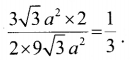Textbook Page No. 75

Class 10 Maths Chapter 3 Kerala Syllabus Question 1.
Raj ani has three necklaces and free pairs of earrings, of green, blue and red stones. In what all different ways can she wear them? What is the probability of her wearing the necklace and earrings of the same color? Of different colors?
Possible ways of wearing:

 Necklace Earring Green Green Green Blue Green Red Blue Green Blue Blue Blue Red Red Green Red Blue Red Red

Raj ani can wear the ornaments in 9 different ways.
Probability of wearing necklace and earring of same colour = $$\frac { 3 }{ 9 }$$ = $$\frac { 1 }{ 3 }$$
Probability of wearing necklace and earring of different colour = $$\frac { 6 }{ 9 }$$ = $$\frac { 2 }{ 3 }$$

Sslc Maths Chapter 3 Notes Question 2.
A box contains four slips numbered 1, 2, 3, 4 and another box contains two slips numbered 1,2. If one slip is taken from each, what is the probability of the sum of numbers being odd? What is the probability of the sum being even?

 First box Second box Sum 1 1 2 1 2 3 2 1 3 2 2 4 3 1 4 3 2 5 4 1 5 4 2 6

Probability of sum being odd = $$\frac { 4 }{ 8 }$$ = $$\frac { 1 }{ 2 }$$
Probability of sum being even = $$\frac { 4 }{ 8 }$$ = $$\frac { 1 }{ 2 }$$

Kerala Syllabus 10th Standard Maths Chapter 3 Question 3.
A box contains four slips numbers 1, 2, 3, 4 and another contains three slips numbered 1, 2, 3. If one slip is taken from each, what is the probability of the product being odd? The probability of the product being even?

 First box Second box Product 1 1 1 1 2 2 1 3 3 2 1 2 2 2 4 2 3 6 3 1 3 3      . 2 6 3 3 9 4 1 4 4 2 8 4 3 12

Probability of sum being odd = $$\frac { 4 }{ 12 }$$ = $$\frac { 1 }{ 3 }$$
Probability of sum being even = $$\frac { 8 }{ 12 }$$ = $$\frac { 2 }{ 3 }$$

10 Th Maths Text Book Questions And Answers Question 4.
From all two-digit numbers with either digit 1, 2 or 3 one number is chosen.
i. What is the probability of both digits being the same?
ii. What is the probability of the sum of the digits being 4?
Two digit numbers
11, 12, 13, 21, 22, 23, 31, 32, 33.
i. P (both digits being same) = $$\frac { 3 }{ 9 }$$ = $$\frac { 1 }{ 3 }$$
ii. P(sum of digits being 4) = $$\frac { 3 }{ 9 }$$ = $$\frac { 1 }{ 3 }$$

10th Class Maths Textbook Answers Question 5.
A game for two players. First, each has to decide whether he wants odd number or even number. Then both raise some fingers of one hand. If the sum is odd, the one who chose odd at the beginning wins; if it is even, the one who chose even wins. In this game, which is the better choice at the beginning, odd or even?
The results in the order when the first player raises one finger and second player raises one finger and so on.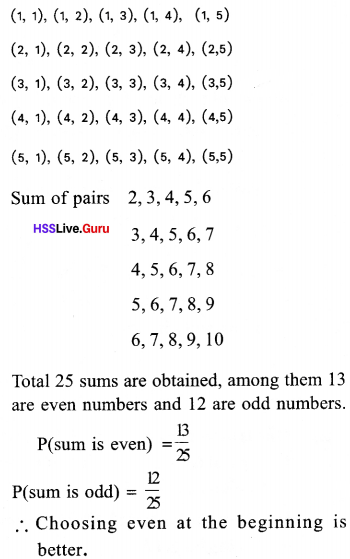Textbook Page No. 78

Maths Chapter 3 Class 10 Kerala Syllabus 10th Standard Question 1.
In class 10A, there are 30 boys and 20 girls. In 10B, there are 15 boys and 25 girls. One student is to be selected from each class.
i. What is the probability of both being girls?
ii. What is the probability of both being boys?
iii. What is the probability of one boy and one girl?
iv. What is the probability of at least one boy?
i. Total no. of possible pairs = 50 × 40 = 2000
No. of pairs in which both are girls = 20 × 25 = 500
Probability of both being girls = $$\frac { 500 }{ 2000 }$$ = $$\frac { 1 }{ 4 }$$

ii No. of pairs in which both are boys = 30 × 15 = 450
Probability of both being boys = $$\frac { 450 }{ 2000 }$$ = $$\frac { 9 }{ 40 }$$

iii. No. of pairs in which one is boy and one is girl = 2000 – (500 + 450) = 2000 – 950 = 1050
Probability of one being boy and one girl = $$\frac { 1050 }{ 2000 }$$ = $$\frac { 21 }{ 40 }$$

iv. No. of pairs in which atleast one is boy = 1050 + 450 = 1500
Probability in which atleast one is boy = $$\frac { 1050 }{ 2000 }$$ = $$\frac { 3 }{ 4 }$$

Question 2.
One is asked to say a two-digit number.
i. What is the probability of both digits being the same?
ii. What is the probability of the first digit being larger?
iii. What is the probability of the first digit being smaller?
i. Probability of two digits being same = $$\frac { 9 }{ 90 }$$ = $$\frac { 1 }{ 10 }$$
(11, 22, 33, 44, 55, 66, 77, 88, 99)

ii. Numbers in which first digit is greater than second digit are 10, 20, 21, 30, 31, 32, 40, 41, 42, 43, 50, 51, 52, 53, 54, 60, 61, 62, 63, 64, 65, 70, 71, 72, 73, 74, 75, 76, 80, 81, 82, 83, 84, 85, 86, 87, 90, 91, 92, 93, 94, 95, 96, 97, 98,
45 outcomes, Required Probability = $$\frac { 45 }{ 90 }$$ = $$\frac { 1 }{ 2 }$$

iii. Numbers in which first digit is smaller than second digit are
12, 13, 14, 15, 16, 17, 18, 19, 23, 24, 25, 26, 27, 28, 29, 34, 35, 36, 37, 38, 39, 45, 46, 47, 48, 49, 56, 57, 58, 59, 67, 68, 69, 78, 79, 89,
36 total no.of favourable outcomes = 36
Probability =Question 3.
Each two-digit number is written on a paper slip and these are all put in a box. What is the probability that the product of the digits of a number drawn is a prime number? What if three-digit numbers are used instead?
Total two-digit numbers = 90
Product of the digits of a number drawn is a prime number 12, 13, 15, 17, 21, 31, 51, 71.
(1 is not a prime number)
Total number whose product of the digits drawn is a prime number = 8
Probability of product of the digits drawn is a prime number = $$\frac { 8 }{ 90 }$$ = $$\frac { 4 }{ 45 }$$
Total three-digit numbers = 900
Product of the digits of a number drawn is a prime number 112, 113, 115, 117, 121, 131, 151, 171.
Total number whose product of the digits drawn is a prime number = 8
Probability of product of the digits drawn is a prime number = $$\frac { 8 }{ 900 }$$ = $$\frac { 2 }{ 225 }$$

Question 4.
Two dice with faces numbered from 1 to 6 are rolled together. What are the possible sums? Which of these sums has the maximum probability?
Each dice has the following numbers: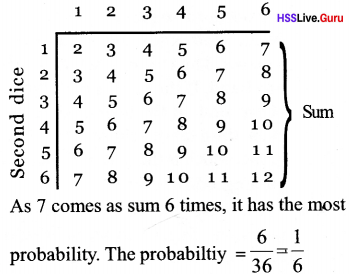### Mathematics of Chance Orukkam Questions & Answers

Worksheet 1

Question 1.
How many odd numbers are there below 25
12 odd numbers

Question 2.
How many prime numbers are there below 30?
10 prime numbers

Question 3.
Find the number of two-digit even numbers?
90 two-digit even numbers

Question 4.
How many two digits perfect squares are there?
6

Question 5.
Write all three-digit numbers that can be written using the digits 3, 6, 8 without repeating the digits.
368, 386, 683, 638, 836, 863

Question 6.
How many multiples of 7 are there in between 100 and 300?
First multiple of 7 = 105
Last multiple of 7 = 294
Number of multiples
=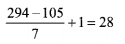Question 7.
There are 50 children in a class. Thirty of them are girls. There are 40 children in another class. 25 of them are boys. One student is taken from each class at random. What is the number of outcomes? How many outcomes contain both boys. How many outcomes contain both girls. How many outcomes have one boy and one girl?
Total pairs = 50 × 40 = 2000
No. of pairs in which both are boys = 20 × 25 = 500
No. of pairs in which both are girls = 30 × 15 = 450
No. of pairs in which one is a boy and the other a girl = 30 × 25 + 20 × 15 = 750 + 300 = 1050

Worksheet 2

Question 8.
A fine dot is placed into the picture with-out looking into it. What is the probability of falling the dot in the small semicircle? What is the probability of falling the dot outside the small semicircle but inside the big semicircle?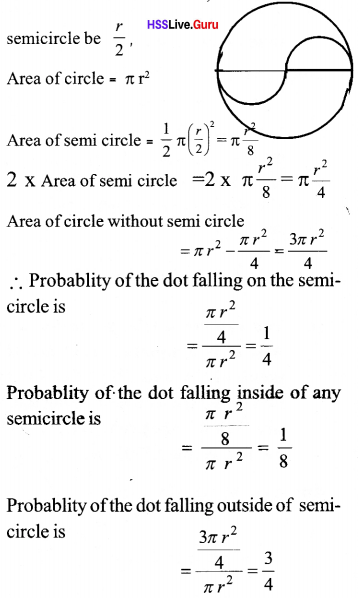Question 9.
P, Q, R are the midpoints of the sides of triangle ABC. Another triangle is drawn by joining these points. A fine dot is placed into the figure without looking into the picture. What is the probability of falling the dot in triangle PQR?
What is the probability of falling the dot outside the triangle?
Area of each small triangle is 1/4th of area of large triangle ABC.Probability of the dot falling on triangle PQR is = 1/4
Probability of the dot falling on small triangle is = 1/4
Probability of the dot falling inside the triangle PQR is $$\frac{3}{4}-\frac{1}{4}=\frac{2}{4}=\frac{1}{2}$$ less than that of outside the triangle.

Question 10.
What is the probability of occurring 53 Sundays in a leap year
Leap year have 366 days.
That is 52 weeks and 2 days.
There two days are
Sunday – Monday, Monday – Tuesday, Tuesday – Wednesday, Wednesday – Thursday, Thursday – Friday, Friday – Saturday, Saturday – Sunday.
∴ Probability for to occur 53 Sunday is 2/7.

Question 11.
You can see a triangle inside a square. ABCD is a square. P, Q are the midpoints of C D and C B. A fine dot is placed into the figure without looking into the figure. What is the probability of falling the dot in triangle APQ?Question 12.
The value of 21, 22, 23… 250 are written in small papers and put it in the box. A paper is taken at random. What is the probability of getting a number having 4 in ones place? What is the probability of falling 8 in the one’s place?
The 13 numbers having 4 in one’s place are 22, 26, 210 …, 250.
∴ Probability = $$\frac { 13 }{ 50 }$$
The 12 numbers having 8 in one’s place are 23, 27, 211 …, 247.
∴ Probability = $$\frac { 12 }{ 50 }$$

Worksheet 3

Question 13.
Numbers from 1 to 10 are written in small papers and placed in a box. One number is taken from the box at random. What is the probability of getting a prime number?
Probability of getting a prime number = $$\frac { 4 }{ 10 }$$ = $$\frac { 2 }{ 5 }$$

Question 14.
Two boxes contain tokens on which numbers 1, 2, 3, 4 are written One token is taken from each box. What is the probability of getting sum of the face numbers a prime number
Pairs of numbers in tokens are
(1.1) , (1,2), (1,3), (1,4)
(2.1), (2, 2), (2, 3), (2, 4)
(3, 1), (3,2), (3; 3), (3,4)
(4, 1), (4, 2), (4, 3), (4,4).
Pairs getting sum as prime numbers
(1.1) , (1,2), (1,4), (2, 1), (2, 3), (3, 2), (3, 4), (4, 1), (4, 3)
Probability of getting sum of the face numbers a prime number = $$\frac { 9 }{ 16 }$$

Question 15.
One box contains 8 black balls and 12 white balls. Another box contains 9 black and 6 white balls. One ball is taken from each box at random. What is probability of getting both black? What is the probability of getting both white? What is the probability of getting one black and one white?
Total pairs = 20 x 15 = 300
Number of pairs getting both black= 8 x 9 = 72
Probability of getting both black = $$\frac { 72 }{ 300 }$$ = $$\frac { 6 }{ 25 }$$
Probability of getting one black and one whiteQuestion 16.
In the figure, a triangle is drawn by joining the alternate vertices of a regular hexagon. A fine dot is placed into the figure at random. What is the probability of falling the dot in the triangle?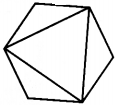Probability of the dot falling on triangle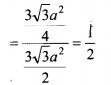Question 17.
What is the probability of occurring four Wednesdays in 23 consecutive days in a month?
23 days = 3 weeks + 2 days
Wednesday comes on Tuesday + Wednesday, Wednesday + Thursday when two days are taken.
Total probabilities (Sunday, Monday), (Monday, Tuesday), (Tuesday, Wednesday), (Wednesday, Thursday), (Thursday, Friday), (Friday, Saturday), (Saturday, Sunday)
∴ Total probabilities = 7
∴ Probabilities = 2/7

### Mathematics of Chance SCERT Question Pool Questions & Answers

Question 18.
One is asked to say a two digit number. What is the probability of being the number not a perfect square? [Score : 3, Time : 3 Minutes]
Total number of two digit number : 90 (1)
Total number of two digit perfect squares: 6 (1)
Number of two digit numbers which are not perfeet squares : 90 – 6 = 84,
Probability = $$\frac { 84 }{ 90 }$$ = $$\frac { 42 }{ 45 }$$ (1)

Question 19.
A bag contains 10 blue balls and 12 yellow balls. Another contains 15 blue balls and 7 yellow balls.
a. What is the probability of getting a yellow ball from the first bag?
b. What is the probability of getting a yellow ball from the second bag?
C. If all the balls are put in a single bag, what is the probability of getting a yellow ball from it? [Score : 4, Time : 4 Minutes]
a. Total number of balls in the first bag = 10 + 12 =22, Number of yellow balls = 12
Probability of getting a yellow ball = $$\frac { 12 }{ 22 }$$ = $$\frac { 6 }{ 11 }$$ (1)

b. Total number of balls in the second bag = 15 + 7 = 22, Number of a yellow ball = 7
Probability of getting a yellow ball = $$\frac { 7 }{ 22 }$$ (1)

c. Total number of balls = 22 + 22 = 44
Number of yellow balls = 12 + 7 = 19 (1)
Probability of getting a yellow ball = $$\frac { 19 }{ 44 }$$ (1)

Question 20.
A regular hexagon is drawn with its vertices on a circle. Without looking into the I picture, if one put dot in that picture, what is the probability of being the dot not in the regular hexagon? [Score: 4, Time: 3 Minutes] .Area of circle = πr2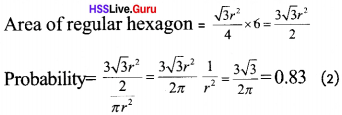Question 21.
In the figure, all the four shaded semicircles have same area. If we put a dot in the figure without looking into it, what is the probability of being the dot in the shaded semicircles? [Score : 3, Time: 5 Minutes]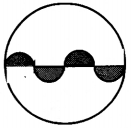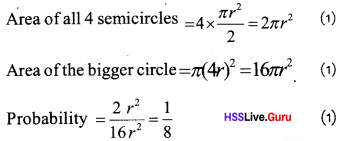Question 22.
What is the probability of getting 5 Sundays in December in a calendar year? [Score : 3, Time : 5 Minutes]
There are 31 days in December. That means 4 full weeks and 3 days.
The probable three days are as shown below.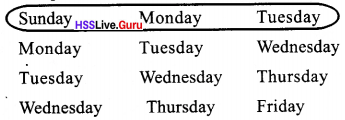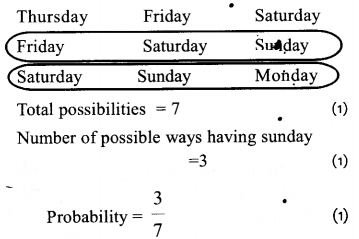Question 23.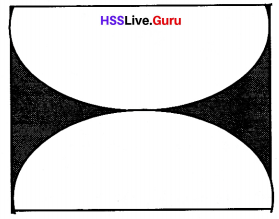Two semicircles are drawn in a square as shown. If we put a dot in the figure, without looking into it, what is the probability of being the dot in the shaded region? [Score: 3, Time: 5 Minutes]
If a is the side of a square,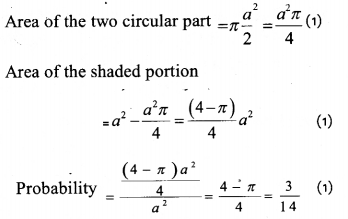### Mathematics of Chance Exam Oriented Questions and Answers

Short Answer Type Questions (Score 2)

Question 24.
A bag contains 6 red balls, 8 green balls, and 8 white balls. One ball is drawn at random from the bag, find the probability of getting
i. A white or green ball
ii. Neither green ball nor a red ball.
Red balls = 6
Green balls = 8
White balls = 8
Total number of balls = 6 + 8 + 8 = 22
a. The probability of getting a white or green ball = 16/20
b. The probability of getting neither green balls nor a red ball = 8/20

Question 25.
20 cards numbered 1, 2, 3, 4, ….19, 20 are put in a box. One boy draws a card from the box. Find the probability that the number on the card is:
i. Prime
ii. Divisible by 3
Total number of outcomes = 20
a. Prime numbers from 1 to 17 are 2, 3, 5, 7, 11,13, 17, 19.
Number of outcomes = 8
The probability that the card drawn is prime number = 8/20

b. Numbers are divisible by 3 are 3, 6, 9, 12, 15, 18.
Number of outcomes = 6
The probability that the card drawn is divisible by 3 = 6/20

Question 26.
In a bag, there were 3 white balls and 5 black balls. From this one ball is taken, then
a. What is the probability of being blackball?
b. What is the probability of being white ball?
Total no. of balls is 8 and in that 5 of then are black balls.
a. Probability of getting black balls = 5/8
b. 3 of the balls were white, so the probability of getting white balls = 3/8

Question 27.
a. How many two-digit natural numbers are there in all?
b. If we choose one number from the two-digit numbers, what is the probability that the sum of digits of that number will be 10?
a. Number of two-digit natural numbers = 90

b. Numbers whose sum of digits will be 10 is 19, 28, 37, 46, 55, 64, 73, 82, 91
Probability that the sum of digits of that number will be 10 = $$\frac { 9 }{ 90 }$$ = $$\frac { 1 }{ 10 }$$

Short Answer Type Questions (Score 3)

Question 28.
In selecting a two-digit number up to 50.
a. What is the probability of the digit in the ten’s place to be larger than the digit in the one’s place?
b. What is the probability of the digit in the tens place to be smaller than the digit in the one’s place?
Total numbers of two-digit numbers up to 50 = 41
a. Number of numbers with the digit in the tens place to be larger than the digit in the units place = 11
Probability that the number with tens place digit is larger than the digit in the unit place = 11/41

b. Probability that the number with the tenth place digit is smaller than the digit in the unit place = 26/41

Question 29.
A black dice and a white dice are thrown at the same time,
a. Write all the possible outcomes.
b. What is the probability that the sum of the two numbers is to be 8.
c. What is the probability that being the same number to be on both dice?
a. Total outcomes
(1,1), (1,2), (1,3), (1,4), (1,5), (1,6) ‘
(2,1), (2,2), (2,3), (2,4), (2,5), (2,6) .
(3,1), (3,2), (3,3), (3,4), (3,5), (3,6)
(4.1), (4,2), (4,3), (4,4), (4,5), (4,6)
(5.1) , (5,2), (5,3), (5,4), (5,5), (5,6)
(6.1), (6,2), (6,3), (6,4), (6,5), (6,6)
Total = 36

b. Sum of two numbers is to be 8 = 5
(2,6), (3,5), (4,4), (5,3), (6,2),
Probability = 5/36

c. Probability of being same number =(1,1),(2,2), (3,3), (4,4), (5,5), (6,6),
Total = 6
Probability = $$\frac { 6 }{ 36 }$$ = $$\frac { 1 }{ 6 }$$

Question 30.
A box contains 400 electronic toy cars. Among them, 12 are defective. One toy is taken out at random. What is the probability that
a. It is a defective toy.
b. It is a non-defective toy.
Total number of cars = 400
Number of defective toys = 12
Number of non-defective toys = 400 – 12 = 388
a. Probability of getting a defective toy = $$\frac { 12 }{ 400 }$$ = $$\frac { 3 }{ 100 }$$

b. Probability of getting a non-defective toy = $$\frac { 388 }{ 400 }$$ = $$\frac { 97 }{ 100 }$$

Long Answer Type Questions (Score 4)

Question 31.
Natural numbers from 1 to 30 are written on paper slips and kept in a box. If one slip is taken from the box,
a. What is the probability of this number to be even?
b What is the probability of this number to be a multiple of 3?
c. What is the probability of this number to be a multiple of 3 and 5?
d. What is the probability that this number be a natural number?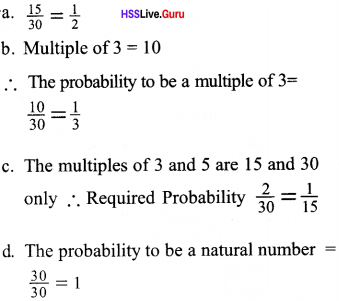Question 32.
There is one spot at one side of the cube, two on another side, three on the third side and so on. There are spots on all the six faces; in this order. Another cube which is marked in the same way is taken.
a. If both the cubes are thrown, what is the probability that the total number of spots on the upper faces is 6?
b. What is the probability that the sum of the spots on the upper faces is 9?
c. What is the probability that the sum of the spots be one?
d. What is the probability that the sum of the spots be a prime number?Question 33.
In a pack of 52 cards, half are red and the rest are black. There are 4 Suits of 13 cards each and having the signs ‘hearts’, ‘spade’, ‘clubs’ and ‘diamond’. If a card is picked from this pack.
a. What is the probability of it being black?
c. What is the probability of it being a spade or a diamond?
a. Total number of cards = 52
Number of black cards = 26
Probability of the picked card being black = $$\frac { 26 }{ 52 }$$ = $$\frac { 1 }{ 2 }$$

b. No. of spade cards = 13
Probability of a picked card being spade = $$\frac { 12 }{ 52 }$$ = $$\frac { 1 }{ 4 }$$

c. No. of cards spade or diamond =13 + 3 = 26
Probability of a picked card being spade or diamond = $$\frac { 26 }{ 52 }$$ = $$\frac { 1 }{ 2 }$$

Long Answer Type Questions (Score 5)

Question 34.
A man is asked to say a 3 digit number,
a. What is the probability that the first and last digits be equal?
b. What is the probability that the last two digits be ‘O’?
c. What is the probability that the last digits being greater than the first?
Total 3 digit numbers = 900
a. Numbers with first and last digits are equal
101, 111, 121, 131, 141, 151, 161, 171, 181, 191, 202, 212, 222, 232, 242, 252, 262, 272, 282, 292, 303, 313, 323, 333, 343, 353, 363, 373, 383, 393
There are 90 such numbers.
∴ Probability = $$\frac { 90 }{ 900 }$$ = $$\frac { 1 }{ 10 }$$

b. The numbers with last two digits zero are 100, 200, 300,……… 900 total numbers 9.
∴ Probability = $$\frac { 9 }{ 900 }$$ = $$\frac { 1 }{ 100 }$$

c. The numbers with the last digit greater than the first digit is 36
∴ probability = $$\frac { 36 }{ 90 }$$ = $$\frac { 2 }{ 5 }$$

Question 35.
There are 10 black pearls and 5 white pearls in the box A. There are 8 black pearls and 7 white pearls in box B.
a. Which box has more probability of be ing the pearls black, when a pearl from each of the boxes is taken?
b. What is the probability to get a white pearl from the box A?
c. What is the probability to get a black pearl from box B?
d. If all the pearls in the box B is dropped in the box A, then what is the probability to get a black pearl from it?
a. Box A because it has more black pearl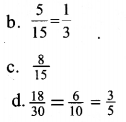Question 36.
Cards marked with the numbers 2 to 101 are placed in a box and mixed thoroughly. One card is drawn from this box. Find the probability that the number of the card is
a. an even number
b. a number less than 16
c. a number which is a perfect square
d. a prime number less than 25